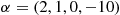Example 5

A simple class of interleavers stems from the following choice of the identity basic permutation:

$\rho \left(i\right)=i-\alpha \left(i\right){N}_{\mathcal{I}}\text{.}$As an example, choosing ${N}_{\mathcal{I}}=4\text{,}\pi =\left(0\text{,}1\text{,}2\text{,}3\right)$, and $\alpha =\left(2\text{,}1\text{,}0\text{,}-10\right)$yields:

$\left[\begin{array}{cccccccccccccccccccc}\hfill i& \hfill \dots & \hfill 0& \hfill 1& \hfill 2& \hfill 3& \hfill 4& \hfill 5& \hfill 6& \hfill 7& \hfill 8& \hfill 9& \hfill 10& \hfill 11& \hfill 12& \hfill 13& \hfill 14& \hfill 15& \hfill 16& \hfill \dots \\ \hfill \rho \left(i\right)& \hfill \dots & \hfill -8& \hfill -3& \hfill 2& \hfill 43& \hfill -4& \hfill 1& \hfill 6& \hfill 47& \hfill 0& \hfill 5& \hfill 10& \hfill 51& \hfill 4& \hfill 9& \hfill 14& \hfill 55& \hfill 8& \hfill \dots \end{array}\right]\text{.}$#### 5.4.2 Implementation of convolutional interleavers with minimal memory requirement

We have ...

Get Channel Coding: Theory, Algorithms, and Applications now with the O’Reilly learning platform.

O’Reilly members experience books, live events, courses curated by job role, and more from O’Reilly and nearly 200 top publishers.